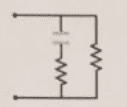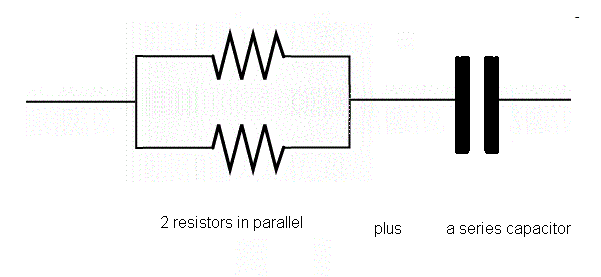# Not sure what I can make into equivalent resistors

• I
• egio

#### egioCould I combine the two resistors together in parallel to make an equivalent resistor, and then use that equivalent resistor in series with the capacitor?

It would be great if there was some rule of thumb that I can use to know what I can make into an equivalent resistor/capacitor down the line once I come across different circuit combinations.

Thanks so much!

Last edited:

Here is the circuit!

Could I combine the two resistors together in parallel to make an equivalent resistor, and then use that equivalent resistor in series with the capacitor?

It would be great if there was some rule of thumb that I can use to know what I can make into an equivalent resistor/capacitor down the line once I come across different circuit combinations.

Thanks so much!
If I understand your questions correctly, the answer is yes to all of them.
Here is a link about calculating the resistance of a resistors wired in parallel:http://www.electronics-tutorials.ws/resistor/res_4.html

If I understand your questions correctly, the answer is yes to all of them.
Here is a link about calculating the resistance of a resistors wired in parallel:http://www.electronics-tutorials.ws/resistor/res_4.html
Ah, I see. So to confirm, I can make an equivalent resistor even though the capacitor is there?

So to confirm, I can make an equivalent resistor even though the capacitor is there?
Yes, as long as the resistors are connected to each other directly.

Yes, as long as the resistors are connected to each other directly.
Hmm.. Can you please clarify what you mean by "directly?"

In order for the resistors to be in parallel, the resistors need to be connected at both ends to the opposite ends of the other resistor(s).

For example, if I have:
* a 10-ohm resistor tied to nodes A and B;
* another 10-ohm resistor tied to nodes A and B; and
* a capacitor tied across nodes A and C (or node A and B).
then those two 10-ohm resistors are equivalent to a single 5-ohm resistor. The same as this:
* a 5-ohm resistor tied to nodes A and B; and
* a capacitor tied across nodes A and C (or node A and B).

On the other hand, if you have this:
* a 10-ohm resistor tied to nodes A and B;
* a 10-ohm resistor tied to nodes A and C; and
* a capacitor tied across nodes B and C
then these resistors are not in parallel and, unless nodes B and C are connected, are not equivalent to 5-ohm (or any other value) resistor.

Hmm.. Can you please clarify what you mean by "directly?"In order for the resistors to be in parallel, the resistors need to be connected at both ends to the opposite ends of the other resistor(s).

For example, if I have:
* a 10-ohm resistor tied to nodes A and B;
* another 10-ohm resistor tied to nodes A and B; and
* a capacitor tied across nodes A and C (or node A and B).
then those two 10-ohm resistors are equivalent to a single 5-ohm resistor. The same as this:
* a 5-ohm resistor tied to nodes A and B; and
* a capacitor tied across nodes A and C (or node A and B).

On the other hand, if you have this:
* a 10-ohm resistor tied to nodes A and B;
* a 10-ohm resistor tied to nodes A and C; and
* a capacitor tied across nodes B and C
then these resistors are not in parallel and, unless nodes B and C are connected, are not equivalent to 5-ohm (or any other value) resistor.
Ah, I see. Thank you for the detailss!

Are you saying that that is what resistors that are connected directly look like? Because then looking at my circuit, the resistors are not connected directly, since the capacitor is in between the upper node that they share. Is my intuition correct?

•Chandra Prayaga
Are you saying that that is what resistors that are connected directly look like? Because then looking at my circuit, the resistors are not connected directly, since the capacitor is in between the upper node that they share. Is my intuition correct?

yes, I am saying my pic is what you should have ... anything else is incorrect for what you described in your OP as what you wanted

Could I combine the two resistors together in parallel to make an equivalent resistor, and then use that equivalent resistor in series with the capacitor?

your image shows that the resistors are NOT in parallel so isn't in line with what you wanted

Dave

yes, I am saying my pic is what you should have ... anything else is incorrect for what you described in your OP as what you wanted

your image shows that the resistors are NOT in parallel so isn't in line with what you wanted

Dave
Oohh I see... If I wanted to find the resistance of the entire circuit, how would I since I can't combine them into an equivalent resistor?

Oohh I see... If I wanted to find the resistance of the entire circuit, how would I since I can't combine them into an equivalent resistor?

way back in post #2, Scott gave you a link to show you how to calculate parallel resistors

http://www.electronics-tutorials.ws/resistor/res_4.html

you can only measure the combined parallel resistance across the ends of the resistors not from the far side of the capacitor
as it will make the resistance look like an open circuit

Oohh I see... If I wanted to find the resistance of the entire circuit, how would I since I can't combine them into an equivalent resistor?
The Equivalent single resistance in the first diagram will depend upon the value of the C and the operating frequency. Taking two extremes: for DC or very low frequencies, the equivalent resistance is the value of the right hand resistor (the C isolates the left hand R completely) and for very high frequencies (where the C behaves like a short circuit), the equivalent resistor is the same as for the two resistors connected in parallel.
For frequencies in between (i.e. one a large range) you need to consider the Impedance of all three circuit elements.